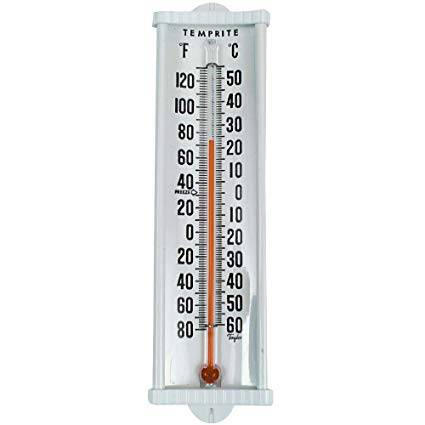# Is 1 degree F > 1degree C ?

• alijan kk
I'm not sure that's true, but in any event, it won't give answer b.Maybe I misunderstood. Comparison of actual temperatures is asked. Try the formula, maybe?.Which of the following is true about the Celsius scale?(a)It is wider than the Fahrenheit scale.(b)It has 100 divisions while the Fahrenheit scale has 180 divisions.(c)It is colder than the Fahrenheit scale.(c)It is colder than the Fahrenheit scale.f

## Homework Statement

which of the following is correct
(a)1oC>1Fo
(b)1Fo>1oC
(c)1Fo=1oCo

## The Attempt at a Solution

F=1.8C+32
change of 1oC is greater than the change of 1oF or I can say it is warmer than change of one degree Fahrenheit.
In the book the answer is b that is 1 F>1C . Is it in any way possible ?

How did you conclude that change of 1 degree C is greater than change of 1 degree F?

How did you conclude that change of 1 degree C is greater than change of 1 degree F?
because there are 100 divisions in centigrade scale and 180 divisions in Fahrenheit scale.

In the book the answer is b that is 1 F>1C . Is it in any way possible ?
Can you post a picture of the problem from your book? You can use the Upload button to upload a PDF or JPEG file...

•SammyS
F=1.8C+32
change of 1oC is greater than the change of 1oF or I can say it is warmer than change of one degree Fahrenheit.
In the book the answer is b that is 1 F>1C . Is it in any way possible ?
They are not asking whether a change in 1 degree on the Celsius scale more than a 1 deg. change on the Fahrenheit scale.

The question is asking whether 1° F is warmer than 1° C.

•Bystander
The question is asking whether 1° F is warmer than 1° C.

I'm not sure that's true, but in any event, it won't give answer b.

I'm not sure that's true, but in any event, it won't give answer b.
Yeah, that's why I edited my Post #4 from saying what Mark44 said, and just asking for clarification of the question. Answer "b" doesn't seem correct, but there could be something else going on with the question...

The question seems pretty unambiguous to me.

The question seems pretty unambiguous to me.
I guess I could be missing the obvious, and we can't give away the solution to homework on the PF, but still...

A degree C is wider than a degree F. And 1 degree C is higher on the thermometer than 1 degree F. So I'm having trouble seeing how "b" could the the solution to the problem... Can you send me a PM to help me out? Thanks!https://images-na.ssl-images-amazon.com/images/I/61Xczv0eYTL._SX425_.jpg#### Attachments

Last edited:
Wait, I've got it finally!

It's asking for which is last alphabetically (higher ASCII representation)! Duh!I'm not sure if you are looking for what ASCII represents or what the actual picture scale of the thermometer represents. You can figure by using or making a graph of F versus C, based on any points of use - like example boiling and freezing points for water. Look at the slope, how 1 unit C and 1 unit F compare. Make Sense?

I'm not sure if you are looking for what ASCII represents or what the actual picture scale of the thermometer represents. You can figure by using or making a graph of F versus C, based on any points of use - like example boiling and freezing points for water. Look at the slope, how 1 unit C and 1 unit F compare. Make Sense?
Sorry, does that lead to selection (b)?

EDIT -- Wait, are you suggesting that we take the derivative with respect to the Kelvin scale? Boy, it seems like we are reaching pretty far to justify what the OP has posted so far...

Last edited:
Sorry, does that lead to selection (b)?

EDIT -- Wait, are you suggesting that wetake the derivative with respect to the Kelvin scale? Boy, it seems like we are reaching pretty far to justify what the OP has posted so far...
My description is not so complicated. Too simple!

## Homework Statement

which of the following is correct
(a)1oC>1Fo
(b)1Fo>1oC
(c)1Fo=1oCo

## The Attempt at a Solution

F=1.8C+32
change of 1oC is greater than the change of 1oF or I can say it is warmer than change of one degree Fahrenheit.
In the book the answer is b that is 1 F>1C . Is it in any way possible ?
Maybe I misunderstood. Comparison of actual temperatures is asked. Try the formula, maybe?.

Choose (a), based on both using the formula, and using the picture of the F and C scales in the picture.

I'm not sure if you are looking for what ASCII represents or what the actual picture scale of the thermometer represents. You can figure by using or making a graph of F versus C, based on any points of use - like example boiling and freezing points for water. Look at the slope, how 1 unit C and 1 unit F compare. Make Sense?
I guess I could be missing the obvious, and we can't give away the solution to homework on the PF, but still...

A degree C is wider than a degree F. And 1 degree C is higher on the thermometer than 1 degree F. So I'm having trouble seeing how "b" could the the solution to the problem... Can you send me a PM to help me out? Thanks!https://images-na.ssl-images-amazon.com/images/I/61Xczv0eYTL._SX425_.jpg
View attachment 229266
so 1 degree is wider than 1 fahrenheit.